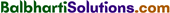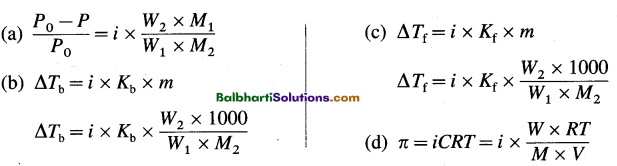By going through these Maharashtra State Board 12th Science Chemistry Notes Chapter 2 Solutions students can recall all the concepts quickly.

## Maharashtra State Board 12th Chemistry Notes Chapter 2 Solutions

→ Henry’s law : S = KHP

→ Raoult’s law : Psoln = x1P0

→ Mole fractions, x1 + x2 = 1

→ Psoln = P°1x1 + P°2x2

→ Psoln = (P°2 – P°1)x2 + P°1→ Mole fractions of components in vapour phase : y1 = $$\frac{x_{1} P_{1}^{0}}{P}$$ and y2 = $$\frac{x_{2} P_{2}^{0}}{P}$$

→ For ideal solutions : ΔVmix = 0; ΔmixH = 0

→ Relative lowering of vapour pressure = $$\frac{P^{0}-P}{P^{0}}$$, x2 = $$\frac{P^{0}-P}{P^{0}}$$

→ $$\frac{P^{0}-P}{P^{0}}=\frac{W_{2} \times M_{1}}{W_{1} \times M_{2}}$$

→ Δ Tb = Kb x m and Δ Tf — Kf x m

→ ΔTb = Kb x $$\frac{W_{2} \times 1000}{W_{1} \times M_{2}}$$

→ ΔTf = Kf x $$\frac{W_{2} \times 1000}{W_{1} \times M_{2}}$$

→ π = cRT and π = $$\frac{W R T}{M \times V}$$

→ i = $$\frac{Colligative property of electrolyte solution}{Colligative property of nonelectrolyte solution of the same concentration}$$

→ Van’t Hoff factor (i) = $$\frac{\Delta T_{\mathrm{b}(\mathrm{ob})}}{\Delta T_{\mathrm{b}(\mathrm{th})}}=\frac{\Delta P_{(\mathrm{ob})}}{\Delta P_{(\mathrm{th})}}=\frac{\Delta T_{\mathrm{f}(\mathrm{ob})}}{\Delta T_{\mathrm{f}(\mathrm{th})}}=\frac{\pi_{\mathrm{ob}}}{\pi_{\mathrm{th}}}=\frac{M_{\mathrm{th}}}{M_{\mathrm{ob}}}$$

→ Colligative properties considering van’t Hoff factor :→ α = $$\frac{i-1}{n-1}$$ (for dissociation)